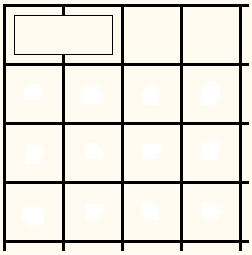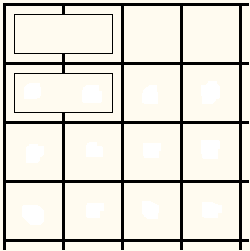# Tiling a Chessboard with Dominoes

Even the simplest of all polyominoes - the domino - affords interesting puzzles. Here is one example [Levitin, #12]:

Is it possible to tile an 8×8 board with dominoes (2×1 tiles) so that no two dominoes form a 2×2 square.

Solution

### References

1. S. Golomb, Polyominoes, Princeton University Press, 1994
2. A. Levitin and M. Levitin, Algorithmic Puzzles, Oxford University Press, 2011
3. G. E. Martin, Polyominoes, MAA, 1996
4. A. V. Shapovalov, The Principle of Narrow Places, MCCME, 2008 (free pdf, in Russian)Is it possible to tile an 8×8 board with dominoes (2×1 tiles) so that no two dominoes form a 2×2 square.

### Solution

The answer is, No, this is impossible, regardless of the board size. To see why, let's just start placing dominoes on the board one at a time and see where this leads us. Assuming, that it is possible to cover the board, a domino will have to be covering a corner square. Because of the symmetry, we may as well assume that the placement is as in the diagram below:The question is where to place the second domino. If the covering is at all possible there have to be dominoes below the one that is already there. Placing it horizontally just below the first one creates a 2×2 square:We are thus forced to place a square vertically:The two dominoes form a corner that needs to be filled. As before, a vertical domino will add up to a 2×2 square, so we are forced to place the third domino horizontally:We get another corner to handle, and, again, a move is forced:So we are forced to continue:Now, reaching the bottom we see that the only way to continue is to create a 2×2 square which shows that the answer to the question at hand is negative. The same process actually shows that tiling of rectangles with one dimension odd and the other even is also impossible unless there is at least one 2×2 square.

Obviously this will happen with any square of even size, while there is no question about tiling an odd square.• Covering A Chessboard With Domino
• Dominoes on a Chessboard
• Vertical and Horizontal Dominoes on a Chessboard
• Straight Tromino on a Chessboard
• Golomb's inductive proof of a tromino theorem
• Tromino Puzzle: Interactive Illustration of Golomb's Theorem
• Tromino as a Rep-tile
• Tiling Rectangles with L-Trominoes
• Squares and Straight Tetrominoes
• Covering a Chessboard with a Hole with L-Trominoes
• Tromino Puzzle: Deficient Squares
• Tiling a Square with Tetrominoes Fault-Free
• Tiling a Square with T-, L-, and a Square Tetrominoes
• Tiling a Rectangle with L-tetrominoes
• Tiling a 12x12 Square with Straight Trominoes
• Bicubal Domino
•